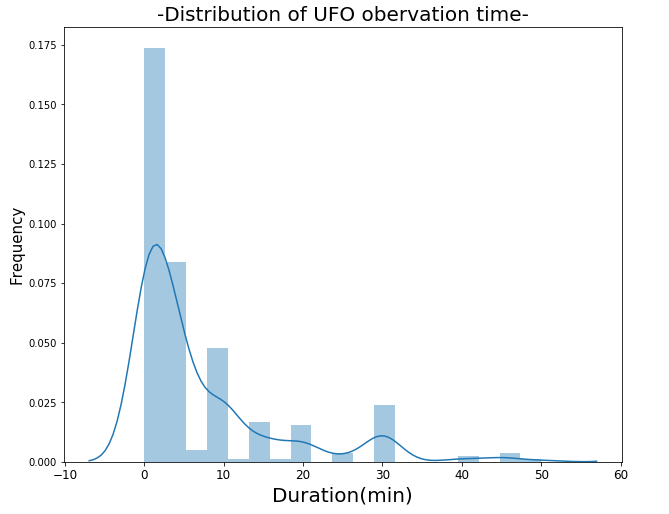﻿ Pandas Datetime: Create a plot of distribution of UFO observation time - w3resource# Pandas Datetime: Create a plot of distribution of UFO observation time

## Pandas Datetime: Exercise-20 with Solution

Write a Pandas program to create a plot of distribution of UFO (unidentified flying object) observation time.

Sample Solution:

Python Code:

``````import pandas as pd
import matplotlib.pyplot as plt
import seaborn as sns
df['duration_sec'] = (df['length_of_encounter_seconds'].astype(float))/60
s = df["duration_sec"].quantile(0.95)
temp = df['duration_sec']
temp = temp.sort_values()
temp = temp[temp < s]
plt.figure(figsize=(10, 8))
sns.distplot(temp)
plt.xlabel('Duration(min)', fontsize=20)
plt.ylabel("Frequency", fontsize=15)
plt.xticks(fontsize=12)
plt.title("-Distribution of UFO obervation time-", fontsize=20)
plt.show()
``````

Sample Output:

```C:\Users\User\Anaconda3\lib\site-packages\scipy\stats\stats.py:1713: FutureWarning: Using a non-tuple sequence for multidimensional indexing is deprecated; use `arr[tuple(seq)]` instead of `arr[seq]`. In the future this will be interpreted as an array index, `arr[np.array(seq)]`, which will result either in an error or a different result.
return np.add.reduce(sorted[indexer] * weights, axis=axis) / sumval```

Python Code Editor:

Have another way to solve this solution? Contribute your code (and comments) through Disqus.

What is the difficulty level of this exercise?

﻿

## Python: Tips of the Day

Returns the symmetric difference between two lists, after applying the provided function to each list element of both

Example:

```def tips_symmetric_difference_by(p, q, fn):
_p, _q = set(map(fn, p)), set(map(fn, q))
return [item for item in p if fn(item) not in _q] + [item for item in q if fn(item) not in _p]
from math import floor
print(tips_symmetric_difference_by([4.2, 2.4], [4.6, 6.8],floor))
```

Output:

```[2.4, 6.8]
```